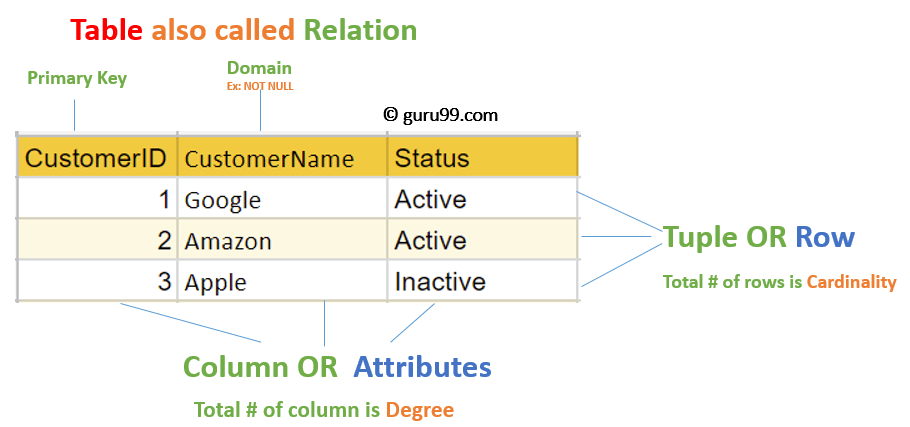The relational model for database management is a method of managing data that employs a structure and language that are consistent with first-order predicate logic. It was first described in 1969 by English computer scientist Edgar F. Codd.

## What is Relational Model?

• Relational Data Model (RM) represents the database as a collection of relations. A relation is nothing but a table of values. Every row in the table represents a collection of related data values. These rows in the table denote a real-world entity or relationship.
• The table and column names aid in interpreting the meaning of the values in each row. The data is represented as a set of relations. Tables are used to store data in the relational model. The physical storage of data, on the other hand, is independent of how the data is logically organized.

Some well-known Relational Database Management Systems are as follows:

• DB2 and Informix Dynamic Server – IBM
• Oracle and RDB – Oracle
• SQL Server and Access – Microsoft

## Basic Terminologies Used in Relational Model of DBMSDiagram identiifying Terminologies Used in Relational Model of DBMS
1. Attribute: Each column in a Table. Attributes are the properties which define a relation. e.g., Student_Rollno, NAME,etc.
2. Tables – In the Relational model the, relations are saved in the table format. It is stored along with its entities. A table has two properties rows and columns. Rows represent records and columns represent attributes.
3. Tuple – It is nothing but a single row of a table, which contains a single record.
4. Relation Schema: A relation schema represents the name of the relation with its attributes.
5. Degree: The total number of attributes which in the relation is called the degree of the relation.
6. Cardinality: Total number of rows present in the Table.
7. Column: The column represents the set of values for a specific attribute.
8. Relation instance – Relation instance is a finite set of tuples in the RDBMS system. Relation instances never have duplicate tuples.
9. Relation key – Every row has one, two or multiple attributes, which is called relation key.
10. Attribute domain – Every attribute has some pre-defined value and scope which is known as the attribute domain

## Operations in Relational Model

Four basic update operations performed on relational database model are

Insert, update, delete and select.

• Insert is used to insert data into the relation
• Delete is used to delete tuples from the table.
• Modify allows you to change the values of some attributes in existing tuples.
• Select allows you to choose a specific range of data.

## Best Practices for creating a Relational Model

• Data need to be represented as a collection of relations
• Each relation should be depicted clearly in the table
• Rows should contain data about instances of an entity
• Columns must contain data about attributes of the entity
• Cells of the table should hold a single value
• Each column should be given a unique name
• No two rows can be identical
• The values of an attribute should be from the same domain